# 10 Must Answer Year 11 Reactive Chemistry Practice Questions

Need to practise your reactive chemistry skills for your yearly exams? Never fear, here are 10 questions to test your knowledge and skills!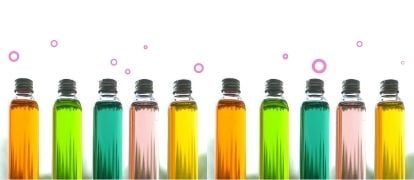Think you’re going well at Chemistry, but can’t be sure? In this post, we give you 10 must answer Year 11 Reactive Chemistry Practice Questions to get you ready for your Yearly exams.

## 10 Must Answer Year 11 Reactive Chemistry Practice Questions

Below are some Module 3 – Reactive Chemistry practice questions to prepare you for your Chemistry yearly exams. Try the questions below, then see how you went against our answers.

### Question 1 – Periodic trends

The following table shows the first and second ionisation energies for Group II metals

 Metal Atomic number 1st Ionisation energy (kJ mol-1) 2nd Ionisation energy (kJ mol-1) Beryllium 4 899 1757 Magnesium 12 738 1450 Calcium 20 590 1145 Strontium 38 549 1064

(a) Define ionisation energy.

(b) Graph ionisation energy against atomic number for both the first ionisation energy and the second ionisation energy. Use the same set of axes for both graphs.

(c) Explain the trend that can be observed in both graphs.

(d) Explain why the first ionisation energy is significantly lower than the second ionisation energy for each of the elements shown in the table.

### Question 2 – Metal reactions

A student reacts three unknown metals with dilute hydrochloric acid.

 Metal Observation X Fast bubbling. Many bubbles form on the metal surface. Y No observable reaction Z Slow bubbling. A few bubbles form on the metal surface

(a) The student is told that the three metals are calcium, zinc and platinum. Identify each of the unknown metals X, Y and Z, and provide reasons for your answer.

(b) Identify the gas produced in the reaction of X and Z with dilute hydrochloric acid. Provide chemical equations to support your answer and suggest a test that could be used to verify the gas produced.

(c) The student reacts samples of X, Y and Z with water and steam. Outline the likely products and provide reasons for your answer.

### Question 3 – Ionic equations

When a 5.0 g sample of magnesium metal is placed in a beaker with sulfuric acid, the mass of the magnesium metal decreases by 1.7 g.

(a) Write a balanced chemical equation for the reaction.

(b) Write a full ionic equation and a net ionic equation for this reaction.

(c) By considering the mass of magnesium that reacted, calculate the volume of gas produced if the reaction took place at 25 oC and 100 kPa.

### Question 4 – Mole questions – percentage composition, empirical formula, molecular formula

A student performed an experiment in which 15.00 g of magnesium was burned in excess oxygen. The product formed had a mass of 24.87 g.

(a) Calculate the mass of oxygen consumed in this reaction.

(b) Calculate the empirical formula of the product.

(c) Explain why the product has an empirical formula but not a molecular formula.

### Question 5 – Mole questions – percentage composition, empirical formula, molecular formula

The percentage composition of a compound is 92.3% carbon and 7.7% hydrogen. The compound is a liquid under standard conditions.

(a) Calculate the empirical formula of this compound.

(b) If the molar mass of this compound is 78.11 g/mol, determine its molecular formula.

(c) The compound reacts with 0.87 mol of chlorine gas to form chlorobenzene ($$C_6H_5Cl_{(l)}$$) and hydrogen chloride gas. Calculate the mass of chlorobenzene produced.

### Question 6 – Limiting reactants

Ammonia is produced by combining nitrogen gas ($$N_2$$) and hydrogen gas ($$H_2$$) at a temperature of 450 oC and pressures of 1.0 atm. How much $$N_2$$ is required to react with an excess $$H_2$$ to produce 20.0 L of ammonia?

### Question 7 – Limiting reactants

A 4.58 g sample of calcium carbonate powder is reacted with 16 mL of 1.0 M hydrochloric acid.

(a) Identify the limiting reactant and calculate the volume of $$CO_2$$ produced at RTP (298 K, 100 kPa).

(b) Calculate the mass of the reactant that remains unreacted.

### Question 8 – Redox

For the following reactions identify the oxidant and reductant.

(a) $$Zn_{(s)} + CuSO_{4(aq)} → ZnSO_{4(aq)} + Cu_{(s)}$$

(b) $$Cl_{2(g)} + Br^{ -}_{(aq)} → 2Cl^{-}_{(aq)} + Br_{2(l)}$$

(c) $$Ca_{(s)} + 2H_2O_{(l)} → Ca(OH)_{2(s)} + H_{2(g)}$$

(d) $$5H_2C_2O_{4(aq)} + 2MnO^{-}_{4(aq)} + 6H^{+}_{(aq)} → 10CO_{2(g)} + 2Mn^{2+}_{(aq)} + 8H_2O_{(l)}$$

### Question 9 – Galvanic and cell potential

An experiment was conducted using two half-cells: One half-cell consisted of 1.0 M $$Zn(NO_{3})_{2}$$ solution with a zinc electrode and the other half-cell consisted of a 1.0 M $$Cu(NO_{3})_{2}$$ solution with a copper electrode. The cells were connected using a salt bridge.

(a) Draw the apparatus described in the experiment and label the anode, cathode, solutions, direction of electron flow, and salt bridge.

(b) Write the equations for both the oxidation and reduction half-cells, and provide the net ionic equation for the process.

(c) Calculate the cell potential of the galvanic cell.

(d) Describe the importance of the salt bridge.

### Question 10 – Rate of reactions

A student conducted an experiment to determine the influence of reactant concentration on reaction rate. The investigation was performed by reacting 0.50 g pellets of aluminium with various concentrations of hydrochloric acid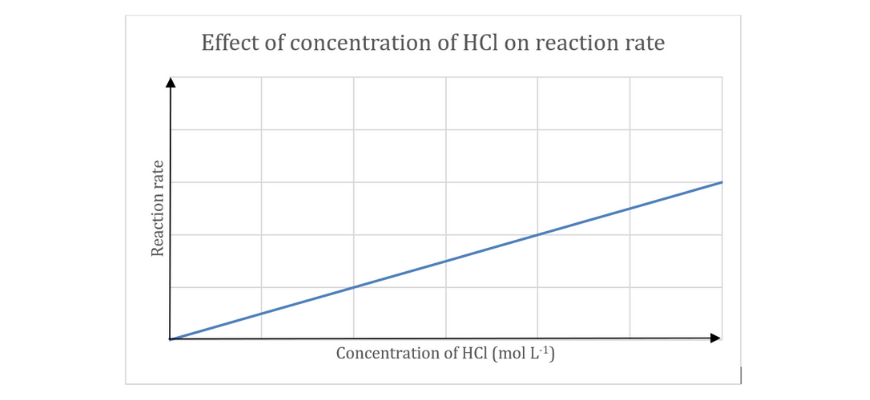(a) Write a balanced chemical reaction for the above reaction.

(b) Identify the independent and dependent variable in this investigation.

(c) With reference to collision theory, explain the trend observed in the graph.

(d) Draw a graph (on the same axes) showing how this graph would be shifted if the investigation was performed at a higher temperature. Give a reason for your answer.

## ANSWERS

### Question 1

(a) Ionisation energy is the energy required to remove one mole of valence electrons from one mole of gaseous atoms from the element.

(b)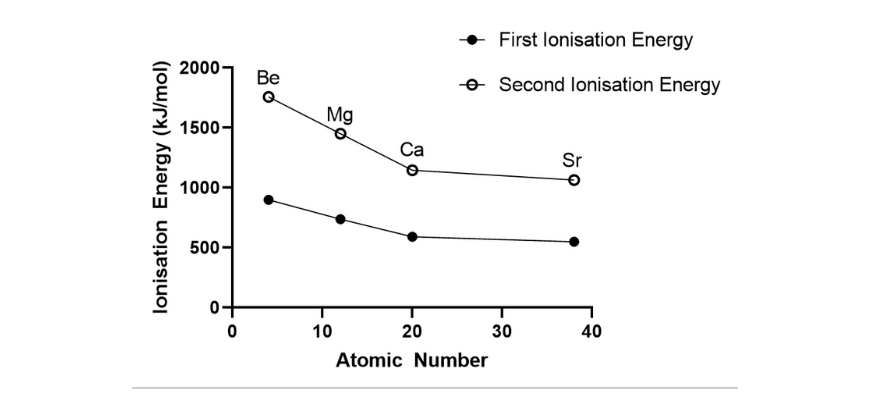(c) From both graphs, it can be observed that as the atomic number increases down a group, both the first and second ionisation energy decreases. Down a group, new shells are added, meaning that the valence electrons are further away from the nucleus and are shielded by inner shell electrons. This results in a reduced attraction between the nucleus and the valence electrons. Hence, less energy is required to remove one mole of valence electrons.

(d) The first ionisation energy involves the removal of one mole of electrons from the valence shell of a neutral atom in the gaseous state, and results in an ion with a 1+ charge. The second ionisation energy is associated with the removal of one mole of electrons from one mole of the ion with a +1 charge. As there are a greater number of protons than electrons in the positively charged ion, there is a greater electrostatic attraction between the valence electrons and the nucleus. As a result, more energy is required to remove the second mole of valence electrons, leading to a higher second ionisation energy.

### Question 2

(a) X – calcium, Y – platinum, Z – zinc. Calcium is higher up the activity series, followed by zinc and then platinum. As the most vigorous reaction was observed for metal X, it must be calcium. As only slow bubbling was observed with metal Z, it must be zinc. Platinum is the least reactive metal and since metal Y did not react, it must be platinum.

(b) Both metals react with HCl to produce hydrogen gas and a chloride salt of the metal
$$Ca_{(s)} + 2HCl_{(aq)} → CaCl_{2{(aq)}} + H_{(g)}$$
$$Zn_{(s)} + 2HCl_{(aq)}→ ZnCl_{2(aq)} + H_{2(g)}$$
The production of hydrogen gas from these reactions can be verified using the pop test.

(c) As calcium is a reactive Group 2 metal, it will react intensely with water to produce calcium hydroxide and hydrogen gas ($$Ca_{(s)} + 2H_2O_{(l)} → Ca(OH)_{2(aq)} + H_{2(g)}$$). Zinc is less reactive than calcium so it will not react with water, but instead will react with steam to produce zinc oxide and hydrogen gas ($$Zn_{(s)} + H_2O_{(g)} → ZnO_{(aq)} + H_{2(g)}$$). Platinum is highly unreactive so will not react with either water or steam.

### Question 3 – Ionic equations

(a) $$Mg_{(s)} + H_2SO_{4(aq)} → MgSO_{4(aq)} + H_{2(g)}$$.

(b) Full ionic equation: $$Mg_{(s)} + 2H^{+}_{(aq)} + SO^{2-}_{4 (aq)} → Mg^{2+}_{(aq)} + SO^{2-}_{4(aq)} + H_{2(g)}$$.
Net ionic equation: $$Mg_{(s)} + 2H^+_{(aq)} → Mg^{2+}_{(aq)} + H_{2(g)}$$

(c) Mass of Mg reacted $$= 5.0 – 1.7 = 3.3 \ g$$.
$$n(Mg) = \frac{3.3}{24.31} = 0.1357 \ mol$$.
$$n(Mg):n(H_2) = 1:1,$$ therefore $$n(H_2) = 0.1357 \ mol$$.
Using the ideal gas law, $$PV = nRT → V = \frac{nRT}{P} = \frac{(0.1357 \times 8.314 \times 298)}{100} = 3.4 \ L$$ (2 sig figs). Alternatively, the equation for molar volume (a simplification of the idea gas law) can be used: $$n = \frac{V}{MV} → V = n \times MV = 0.1357 \times 24.79 = 3.4 \ L {(2 sig.figs)}$$

### Question 4 – Mole questions

(a) Mass of oxygen $$= 24.87 – 15.00 = 9.87 \ g$$ (2 decimal places.) (Note: decimal places are used to determine the number of digits given for values determined by subtraction and addition. Read our article on significant figures for more information)

(b) The product contains $$Mg$$ and $$O$$.
$$n(Mg) = \frac{15.00}{24.31} = 0.617 \ mol$$.
$$n(O2) = \frac{9.87}{16.00} = 0.617 \ mol$$.
$$n(Mg):n(O) = 1:1$$, therefore the empirical formula of the product is $$MgO$$.

(c) $$MgO$$ is an ionic compound which exists in a lattice structure and not as discrete molecules. Hence, its formula is the simplest ratio of its component elements and not a molecular formula.

### Question 5 – Mole questions – percentage composition, empirical formula, molecular formula

(a) The empirical formula for a compound can be easily calculated by assuming that the sample has a mass of 100 g, meaning that the percentages of each element correspond to the mass of each element. For this question, this means that the compound would consist of 92.3 g of carbon and 7.7 g of hydrogen. From here, the number of moles of each component is calculated, and the simplest mole ratio determined:

$$n(C) = \frac{92.3}{12.01} = 7.68 \ mol$$
$$n(H) = \frac{7.7}{1.008} = 7.64 \ mol$$.
$$n(C): n(H) = 1:1$$.
Therefore, the empirical formula is $$CH$$.

(b) number of empirical formula units $$(x) = \frac{\text{molar mass }(MM)}{\text{empirical formula mass}} = \frac{78.11}{12.01+1.008} = 6$$. Therefore, the formula is $$(CH)_6 = C_6H_6$$

(c) $$C_6H_{6(l)} + Cl_{2(g)} → C_6H_5Cl_{(l)} + HCl_{(g)}.$$
$$n(C_6H_5Cl) = n(Cl_2) = 0.87 \ mol$$
$$m(C_6H_5Cl) = 0.87 \times ((6 \times 12.01) + (5 \times 1.008) + 35.45) = 97.9185 = 98 \ g \text{(2 sig figs)}$$.

### Question 6 – Limiting reactants

$$N_{2(g)} + 3H_{2(g)} → 2NH_{3(g)}$$

Number of moles of $$NH_3$$:

$$PV = nRT → n = \frac{PV}{RT} = \frac{(1.0 \times 20.0)}{(8.314 \times 723)} = 0.00332722122 \ mol$$
$$n(NH_3):n(N_2) = 2:1$$, therefore $$n(N_2) = \frac{00332722122}{2} = 0.00166361061 \ mol$$
$$m = 0.00166361061 \times (14.01 \times 2) = 0.047 \ g \ \text{(2 sig figs)}$$.

### Question 7 – Limiting reactants

(a) $$CaCO_{3(s)} + 2HCl_{(aq)} → CaCl_{2(aq)} + CO_{2(g)} + H_2O_{(l)}$$.

$$n(CaCO_3) = \frac{4.58}{(40.08 + 12.01 + (3 \times 16.00))} = 0.0457588 \ mol$$
$$n(HCl) = cV = 0.016 \times 1.0 = 0.016 \ mol$$

Therefore, the limiting reactant is $$HCl$$.

$$n(HCl): n(CO_2) = 2:1$$, so $$n(CO_2) = 0.0080 \ mol$$.

The volume of gas can be found using the ideal gas law:

$$PV = nRT → V = \frac{nRT}{P} = (0.0080 \times 8.314 \times 298){100} = 0.19 \ L \ \text{(2 s.f.)}$$.

(b) $$CaCO_3$$ is in excess
$$CaCO_3 \text{ reacted} = 0.0080 \ mol$$
$$m(CaCO_3 \text{ reacted}) = 0.0080 \times (40.08 + 12.01 + (3 \times 16.00)) = 0.80072 \ g$$.
Hence, unreacted $$CaCO_3 = 4.58 – 0.80072 = 3.8 \ g \text{(2 s.f.)}$$

### Question 8 – Redox

(a) $$Cu^{2+}$$ is the oxidant, $$Zn$$ is the reductant

(b) $$Cl_2$$ is the oxidant, $$Br^-$$ is the reductant

(c) $$H^+$$ is the oxidant, $$Ca$$ is the reductant

(d) $$MnO^{-}_{4}$$ is the oxidant, $$H_2C_2O_4$$ is the reductant

### Question 9 – Galvanic and cell potential

(a)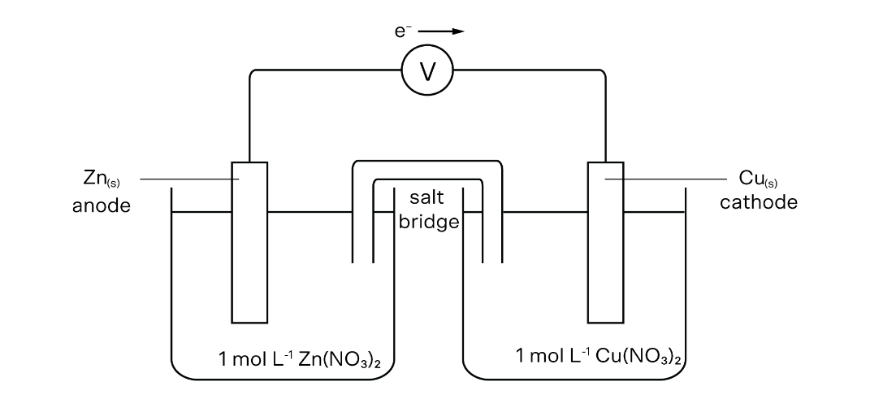(b)
Oxidation: $$Zn_{(s)} → Zn^{2+} + 2e^-$$
Reduction: $$Cu^{2+} + 2e^- → Cu_{(s)}$$
Net ionic equation: $$Zn_{(s)} + Cu^{2+} → Zn^{2+} + Cu_{(s)}$$

(c) $$E_{\text{cell}} = 0.76 + 0.34 = 1.10 \ V$$

(d) The salt bridge completes the circuit and prevents the build-up of charge.

### Question 10 – Rate of reactions

(a) $$2Al_{(s)} + 6HCl_{(aq)} → 2AlCl_{3(aq)} + 3H_{2(g)}$$

(b) The independent variable is the concentration of hydrochloric acid, and the dependent variable is the reaction rate.

(c) According to collision theory, the reaction rate is dependent on the frequency of effective collisions. An effective collision occurs when reactant molecules collide with sufficient energy (greater than or equal to the activation energy) and correct orientation. In this investigation, as the concentration of hydrochloric acid increases, the likelihood of an effective collision between hydrochloric acid and aluminium increases, thereby increasing the rate of reaction.

(d) As temperature increases, the kinetic energy of the particles also increases. As a result, reactant particles collide with a higher frequency and a higher average kinetic energy. Thus, the number of effective collisions increases, leading to an increased reaction rate.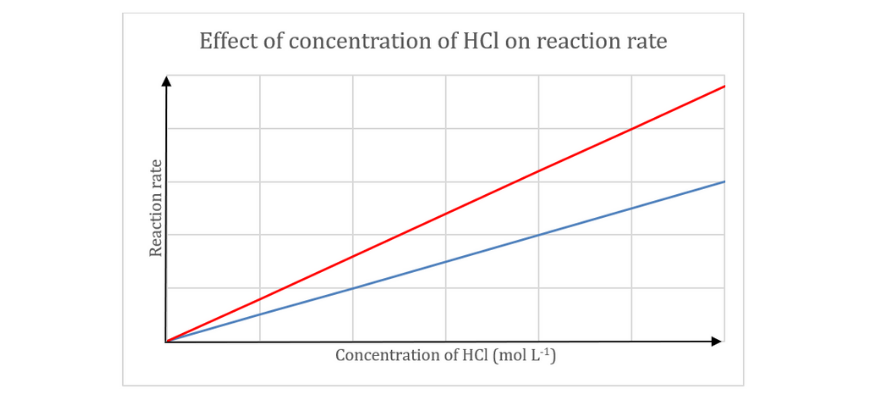## Want to increase your Chemistry marks?

Learn from the best, online, with Matrix+ Chemistry courses! Learn more.### Get free study tips and resources delivered to your inbox.

Join 75,893 students who already have a head start.

Our website uses cookies to provide you with a better browsing experience. If you continue to use this site, you consent to our use of cookies. Read our cookies statement.